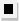Chapter 13.CR, Problem 60E### Algebra and Trigonometry (MindTap ...

4th Edition
James Stewart + 2 others
ISBN: 9781305071742

#### Solutions

Chapter
Section### Algebra and Trigonometry (MindTap ...

4th Edition
James Stewart + 2 others
ISBN: 9781305071742
Textbook Problem

# 5 5 - 6 0Infinite Geometric Series Determine whether the infinite geometric series is convergent or divergent. If it is convergent, find its sum. a + a b 2 + a b 4 + a b 6 ⋅ ⋅ ⋅ , | b | < 1

To determine

Whether the infinite geometric series a+ab2+ab4+ab6, |b|<1 is convergent or divergent. Find the sum if convergent.

Explanation

Given:

The expression is given as,

a+ab2+ab4+ab6, |b|<1

Approach:

Check if the absolute value of the common ratio is smaller than 1. If convergent use the formula for sum of an infinite series.

Calculation:

The common ratio is,

r=ab2a

### Still sussing out bartleby?

Check out a sample textbook solution.

See a sample solution

#### The Solution to Your Study Problems

Bartleby provides explanations to thousands of textbook problems written by our experts, many with advanced degrees!

Get Started